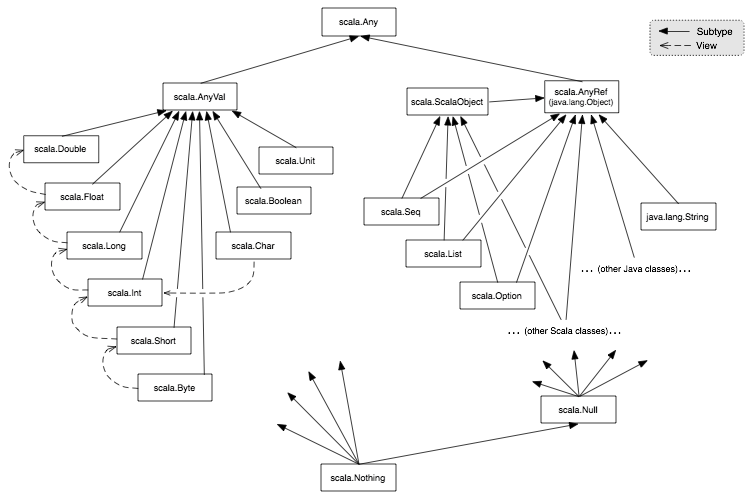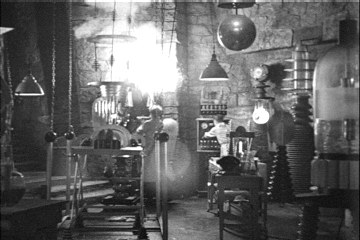# Programmation appliquée en ScalaCopyright © Cay S. Horstmann 2015# Generic Types and Functions

• Generic type has type parameters. Example: `Map[K, V]`
• Instantions of type `Map[String, Int]`, `Map[Person, Person]`, ...
• Classes and traits can be generic
• Generic function has type parameters. Example:
```def getMiddle[T](a: Vector[T]) = a(a.length / 2)
```
• Instantiations of function `getMiddle[Int]`, `getMiddle[String]`, ...
• Functions and methods can be generic

# Upper Bounds

• Consider `Pair` type where both components have the same type:
```class Pair[T](val first: T, val second: T)
```
• Add method to produce the smaller value:
```class Pair[T](val first: T, val second: T) {
def smaller = if (first.compareTo(second) < 0) first else second // Error
}
```
• That can't work—not every `T` has a `comparable` method
• Remedy: Specify an “upper bound”
`class Pair[T <: Comparable[T]]`
• `T` must be a subtype of `Comparable[T]`

# Super- and Subtypes

• In the theory of types, have relationships between types S and T.
• Intuitively, S is a subtype of T, if you can provide a value of type S whenever a type T is expected.
• Notation S `<:` T
• Example: If the class `Student` extends `Person`, then `Student <: Person`
• Example: `Vector[Int] <: Seq[Int]`
• If S is a subtype of T, then T is a supertype of S
• Is `Int` a subtype of `Double`? Not in Scala—but there is an automatic conversion from `Int` to `Double`.

# Scala Type Hierarchy# How Many Statements are Correct?

1. `Null` is a subtype of all Scala types
2. `Nothing` is a supertype of all Scala types
3. `Unit` is a subtype of all Scala types
4. An instance of any Scala type can be converted to `Unit`
1. None of them is correct
2. One is correct of them is correct
3. Two are correct
4. All are correct

# Lower Bounds

• Consider `Pair` class with `replaceFirst` method:
```class Pair[T](val first: T, val second: T) {
def replaceFirst(newFirst: T) = new Pair[T](newFirst, second)
}```
Note that `replaceFirst` returns a new object—`Pair` is immutable
• Suppose we have a `Pair[Student]` and a `Person` object. Can we call
`students.replaceFirst(person)`
• Sure, but the result should be a `Pair[Person]`.
• In general, the replacement type can be a supertype of `T`.
• Use lower bound:
```def replaceFirst[R >: T](newFirst: R) = new Pair[R](newFirst, second)
```
• Note: The return type can be inferred:
```def replaceFirst[R >: T](newFirst: R) = new Pair(newFirst, second)
```

# Context Bounds

• A context bound requires that there is an “implicit value” for a type. For example,
`class Pair[T : Ordering]`
requires an implicit value of type `Ordering[T]`
• `Ordering[T]` defines a method `compare(T, T)`
• Suppose we want a `Pair[Int]`
• “Somewhere”, there must be a definition
```implicit object NameDoesNotMatter extends Ordering[Int] {
def compare(a: Int, b: Int) = if (a < b) -1 else if (a > b) 1 else 0
}```
• `implicitly` fetches implicit object:
```class Pair[T : Ordering](val first: T, val second: T) {
def smaller =
if (implicitly[Ordering[T]].compare(first, second) < 0) first else second
}```

# Lab• You work with a buddy
• One of you (the coder) writes the code, the other (the scribe) types up answers
• When you get stuck, ask your buddy first!
• Switch coder/scribe roles each lab
• The coder submits the worksheet. Include the scribe's name in the worksheet!
• The scribe submits answers. Include the coder's name in the report!

# Part 1: Upper Bounds

1. Using classes
```class Person(val name: String)
class Student(name: String, val major: String) extends Person(name)
```
and the `Pair` class from the “Lower Bounds” slide
```class Pair[T](val first: T, val second: T) {
def replaceFirst[R >: T](newFirst: R) = new Pair(newFirst, second)
}
```

make a `Pair[Student]`, and then replace the first element with a `Person` object. What is the type of the result? Why?

2. Now do the opposite, making a `Pair[Person]`, and replacing the first element with a `Student` object. What is the type of the result? Why?
3. Now remove the upper bound in the `replaceFirst` method:
```def replaceFirst[R](newFirst: R) = new Pair(newFirst, second)
```
Note that the method still compiles. Repeat the preceding experiments, replacing the first element in a `Pair[Student]` and `Pair[Person]`. Which of them still work? Pay close attention to the types of the returned pairs!

# Part 2: Generic Functions

1. Define a generic function `swap` that swaps the components of a 2-tuple. For example, `swap((2, "Hi"))` should be `("Hi", 2)`
2. Define a generic function `sort` that sorts the components of a 2-tuple of type `(T, T)` (where both components have the same type). Require the type to implement the `Comparable` interface. Hint: Swap them if they are not in order. For example, `sort(("Hi", "Bye"))` should be `("Bye", "Hi")`.
3. Define a generic function `sort` that sorts the components of a 2-tuple of type `(T, T)`, given a comparison function. For example,
`sort(("Hi", "Bye"), (s: String, t: String) => s.length - t.length)`
4. What happens when you call
`sort((4, 3), _ - _)`
5. Fix that with a curried generic function `csort` so that `csort((4, 3))(_ - _)` works.

# Part 3: View Bounds

1. What happens when you call the `sort` method from Part 2 with the pair `(4, 3)`?
2. The trouble is that `Int` does not implement `Comparable[Int]`. It's actually not easy to verify that. But try this:
```val x1 = 3
x1.getClass
val x2: Comparable[Int] = 3
x2.getClass
```
What are the classes of `x1` and `x2`?
3. Integers are automatically converted to `RichInt` when needed. In the Scaladoc of `RichInt`, click on “Linear Supertypes”. Note that `Comparable[Int]` is a supertype. So, the following should work:
```import scala.runtime.RichInt
sort((new RichInt(4), new RichInt(3)))```
But it doesn't. Why? (Hint: What is `T` in this case? What is `Comparable[T]`? Is `T` a subtype of `Comparable<T>`?)
4. This is a common problem, caused by the multitude of automatic conversions that are needed to make Scala work with Java types. The remedy is to use a weaker relationship than subtyping, written as S `<%` T, to indicate that S can be implicitly converted to a subtype of T. In the definition of `sort`, change `<:` to `<%`. Can you now sort a pair of `Int`? A pair of `RichInt`? Explain why.
5. The `Comparable` interface is used in Java. In Scala, we prefer `Ordered`. Consider this method:
```def osort[T <: Ordered[T]](pair: (T, T)) =
if (pair._1 < pair._2) pair else swap(pair)```
What happens if you call `osort(("Hi", "Bye"))`?
6. Why does that happen? (Hint: Find out what a `java.lang.String` is automatically converted to when an `Ordered[String]` is needed.)
7. How do you fix it?

# Part 4: Context Bounds

1. The `<%` trick is a bit icky, and it's no longer recommended. Instead, use a context bound. Complete the following function, using the same logic as in the “Context Bounds” slide.
`def psort[T : Ordering](pair: (T, T)) = ...`
2. Verify that `psort` works for pairs of strings and integers. What did you try?
3. Now add this class to the worksheet:
```class Point(val x: Double, val y: Double) {
override def toString = s"(\${x},\${y})"
}```
4. What happens when you pass two `Point` objects to `psort`?
5. Add a definition
```implicit object NameDoesNotMatter extends Ordering[Point] {
def compare(a: Point, b: Point) = ...
}```
6. and define comparison of points in some way of your choice. What happens to the call to `psort` in the preceding step?